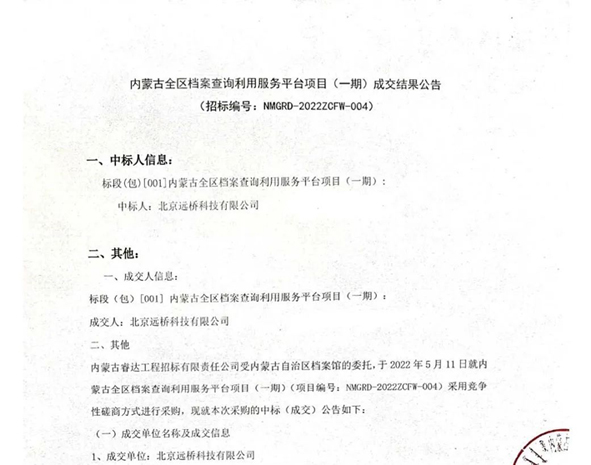##### 远桥“虎”力全开，捷报频传！

<span style="color: rgb(2, 30, 170); font-family: -apple-system, BlinkMacSystemFont, " helvetica="" neue",="" "pingfang="" sc",="" "hiragino="" sans="" gb",="" "microsoft="" yahei="" ui",="" yahei",="" arial,="" sans-serif;="" font-size:="" 17px;="" font-weight:="" 700;="" letter-spacing:="" 0.544px;="" text-align:="" justify;="" background-color:="" rgb(255,="" 255,="" 255);="" text-decoration:="" underline;"="">    远桥科技中标 “ 内蒙古全区档案查询利用服务平台项目（一期）”